# Examples

## IntroductionThis vignette shows some examples how to set up different inference models in babette.

For all examples, do load babette:

library(babette)

All these examples check that BEAST2 is installed at the default location at /home/richel/.local/share/beast/lib/launcher.jar. If this is not the case, we will use some fabricated output:

posterior <- create_test_bbt_run_output()
posterior$anthus_aco_sub_trees <- posterior$anthus_aco_trees
names(posterior)
#>  "estimates"            "anthus_aco_trees"     "operators"
#>  "output"               "anthus_aco_sub_trees"

All examples read the alignment from a FASTA file (usually my_fasta.fas).

fasta_filename <- get_babette_path("anthus_aco_sub.fas")

Instead of a full run, the MCMC chain length is shortened to 10K states, with a measurement every 1K states:

mcmc <- create_test_mcmc(chain_length = 10000)

We will re-create this MCMC setup, as doing so initializes it with new filenames for temporary files. These temporary files should not exist before a run and should exist after a run. Sure, there is the option to overwrite…

## Example #1: all default

Using all default settings, only specify a DNA alignment.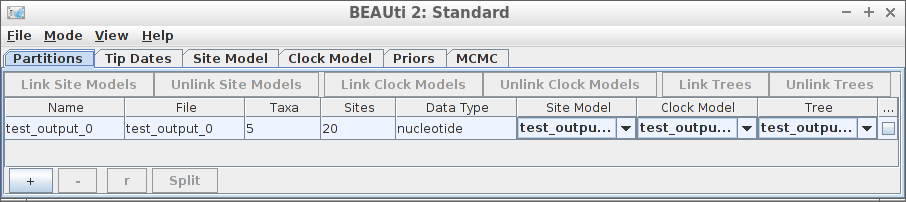Example #1: all default

if (is_beast2_installed()) {
inference_model <- create_inference_model(
mcmc = mcmc
)
beast2_options <- create_beast2_options()
posterior <- bbt_run_from_model(
fasta_filename = fasta_filename,
inference_model = inference_model,
beast2_options = beast2_options
)
bbt_delete_temp_files(
inference_model = inference_model,
beast2_options = beast2_options
)
}

All other parameters are set to their defaults, as in BEAUti.

plot_densitree(posterioranthus_aco_sub_trees, width = 2)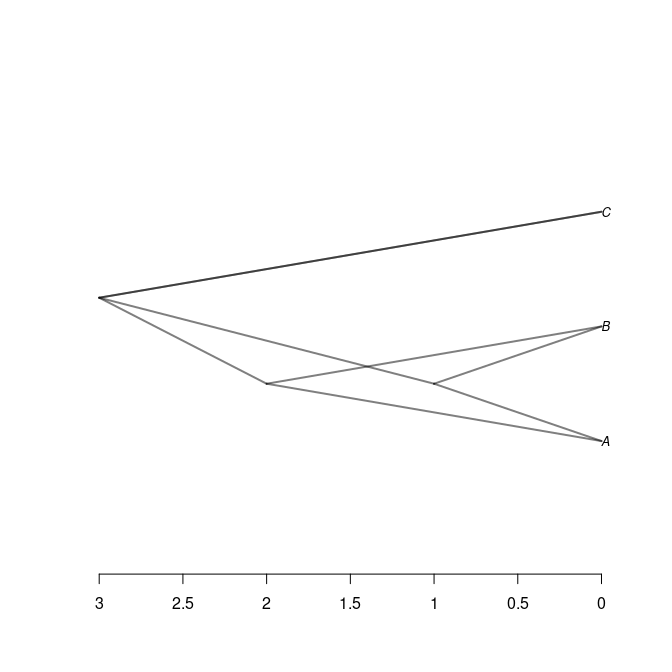## Example #2: using an MRCA prior to specify a crown age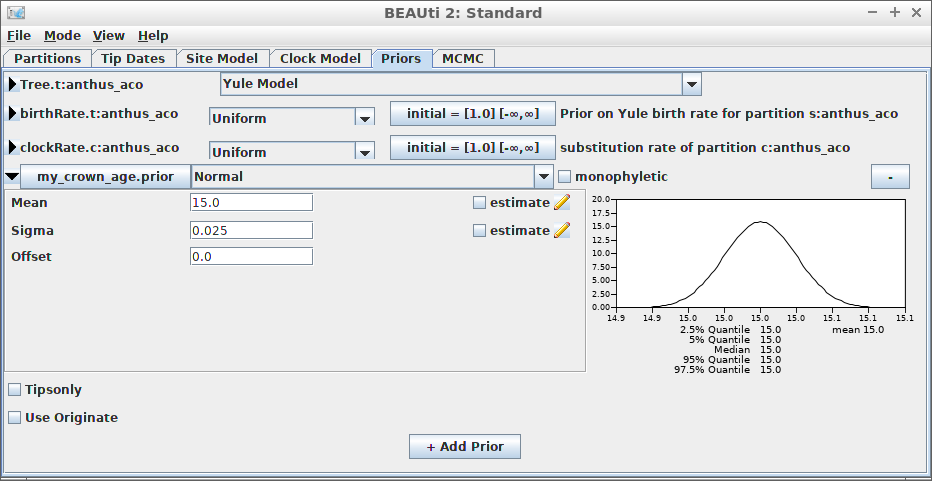Example #2: using an MRCA prior to specify a crown age An alternative is to date the node of the most recent common ancestor of all taxa. Create the MCMC: mcmc <- create_test_mcmc(chain_length = 10000) if (is_beast2_installed()) { inference_model <- create_inference_model( mcmc = mcmc, mrca_prior = create_mrca_prior( taxa_names = sample(get_taxa_names(fasta_filename), size = 3), alignment_id = get_alignment_id(fasta_filename), is_monophyletic = TRUE, mrca_distr = create_normal_distr( mean = 15.0, sigma = 0.025 ) ) ) beast2_options <- create_beast2_options() posterior <- bbt_run_from_model( fasta_filename = fasta_filename, inference_model = inference_model, beast2_options = beast2_options ) bbt_delete_temp_files( inference_model = inference_model, beast2_options = beast2_options ) } Here we use an MRCA prior with fixed (non-estimated) values of the mean and standard deviation for the common ancestor node’s time. plot_densitree(posterioranthus_aco_sub_trees, width = 2)## Example #3: JC69 site model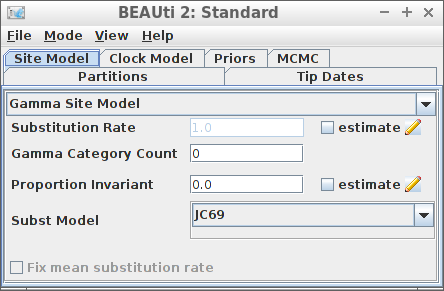Example #3: JC69 site model

if (is_beast2_installed()) {
inference_model <- create_inference_model(
site_model = create_jc69_site_model(),
mcmc = mcmc
)
beast2_options <- create_beast2_options()
posterior <- bbt_run_from_model(
fasta_filename = fasta_filename,
inference_model = inference_model,
beast2_options = beast2_options
)
bbt_delete_temp_files(
inference_model = inference_model,
beast2_options = beast2_options
)
}
plot_densitree(posterior$anthus_aco_sub_trees, width = 2)## Example #4: Relaxed clock log normal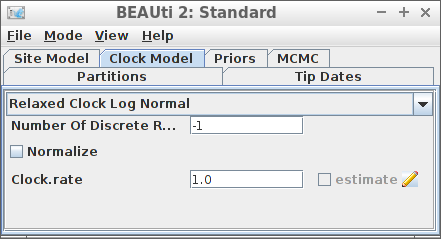Example #4: Relaxed clock log normal if (is_beast2_installed()) { inference_model <- create_inference_model( clock_model = create_rln_clock_model(), mcmc = mcmc ) beast2_options <- create_beast2_options() posterior <- bbt_run_from_model( fasta_filename = fasta_filename, inference_model = inference_model, beast2_options = beast2_options ) bbt_delete_temp_files( inference_model = inference_model, beast2_options = beast2_options ) } plot_densitree(posterior$anthus_aco_sub_trees, width = 2)## Example #5: Birth-Death tree prior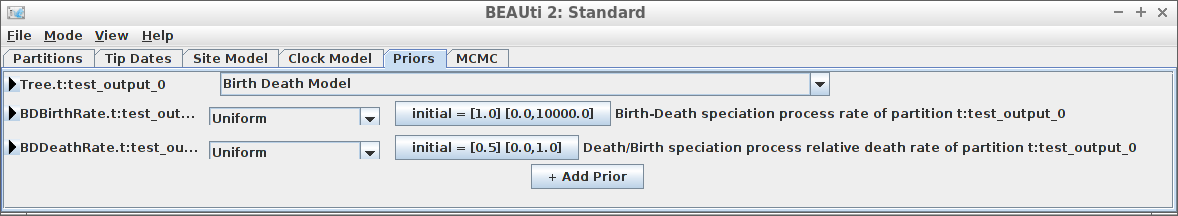Example #5: Birth-Death tree prior

if (is_beast2_installed()) {
inference_model <- create_inference_model(
tree_prior = create_bd_tree_prior(),
mcmc = mcmc
)
beast2_options <- create_beast2_options()
posterior <- bbt_run_from_model(
fasta_filename = fasta_filename,
inference_model = inference_model,
beast2_options = beast2_options
)
bbt_delete_temp_files(
inference_model = inference_model,
beast2_options = beast2_options
)
}
plot_densitree(posterior$anthus_aco_sub_trees, width = 2)## Example #6: Yule tree prior with a normally distributed birth rate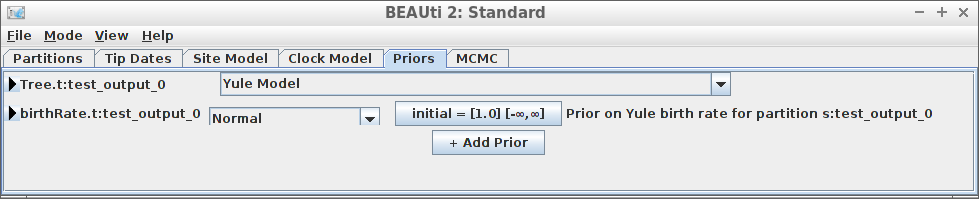Example #6: Yule tree prior with a normally distributed birth rate if (is_beast2_installed()) { inference_model <- create_inference_model( tree_prior = create_yule_tree_prior( birth_rate_distr = create_normal_distr( mean = 1.0, sigma = 0.1 ) ), mcmc = mcmc ) beast2_options <- create_beast2_options() posterior <- bbt_run_from_model( fasta_filename = fasta_filename, inference_model = inference_model, beast2_options = beast2_options ) bbt_delete_temp_files( inference_model = inference_model, beast2_options = beast2_options ) } plot_densitree(posterior$anthus_aco_sub_trees, width = 2)Thanks to Yacine Ben Chehida for this use case

## Example #7: HKY site model with a non-zero proportion of invariants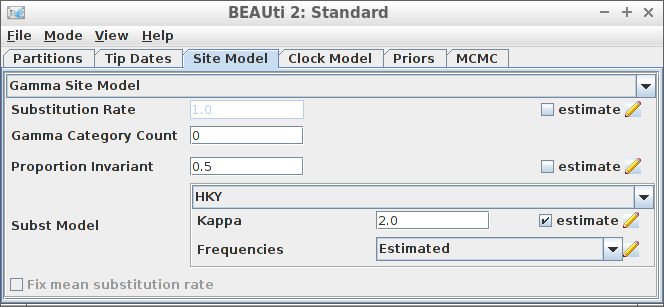Example #7: HKY site model with a non-zero proportion of invariants

if (is_beast2_installed()) {
inference_model <- create_inference_model(
site_model = create_hky_site_model(
gamma_site_model = create_gamma_site_model(prop_invariant = 0.5)
),
mcmc = mcmc
)
beast2_options <- create_beast2_options()
posterior <- bbt_run_from_model(
fasta_filename = fasta_filename,
inference_model = inference_model,
beast2_options = beast2_options
)
bbt_delete_temp_files(
inference_model = inference_model,
beast2_options = beast2_options
)
}
plot_densitree(posterior$anthus_aco_sub_trees, width = 2)Thanks to Yacine Ben Chehida for this use case ## Example #8: Strict clock with a known clock rate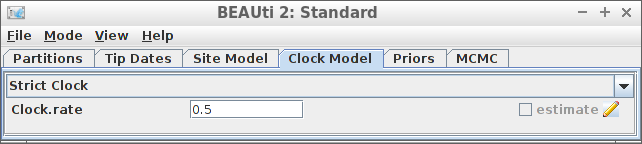Example #8: Strict clock with a known clock rate if (is_beast2_installed()) { inference_model <- create_inference_model( clock_model = create_strict_clock_model( clock_rate_param = 0.5 ), mcmc = mcmc ) beast2_options <- create_beast2_options() posterior <- bbt_run_from_model( fasta_filename = fasta_filename, inference_model = inference_model, beast2_options = beast2_options ) bbt_delete_temp_files( inference_model = inference_model, beast2_options = beast2_options ) } plot_densitree(posterior$anthus_aco_sub_trees, width = 2)Thanks to Paul van Els and Yacine Ben Chehida for this use case.